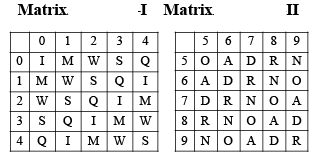Directions : In the following question, a word is represented by only one set of numbers as given in any one of the alternatives.  The sets of numbers given in the alternatives are represented by two classes of alphabets as in the two matrices given below.  The columns and rows of Matrix I and numbered from 0 to 4 and that of Matrix II and numbered from 5 to 9.  A letter from these matrices can be represented first by its row and next by its column, e.g. ‘M’ can be represented by 01, 10, etc.  and ‘A’ can be represented by 56, 65 etc.  Similarly, you have to identify the set for the word given in each question.• a)
56, 66, 86
• b)
58, 69, 65
• c)
58, 66, 78
• d)
67, 96, 57Madhu Tomar Apr 21, 2022